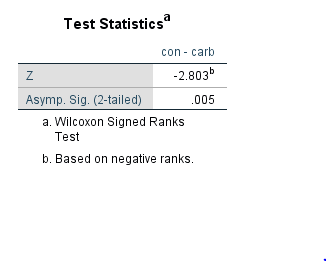#### Howdy, Stranger!

It looks like you're new here. If you want to get involved, click one of these buttons!

Supported by

# Wilcoxon signed rank test - Jasp vs Python, slightly different p values

I ran Wilcoxon signed test using scipy.stats.wilcoxon (https://docs.scipy.org/doc/scipy/reference/generated/scipy.stats.wilcoxon.html) and Jasp.

I got p=0.005 in python and p=0.002 in Jasp, I was wondering why might that be?

I attach a csv file with the paired data I was testing.

• I'll pass this on to Johnny. We use a particular R package I think.
Cheers,
E.J.

• Hi Alebyk,
The difference lies in whether a normal approximation is used for the p-value. From the SciPy page: "Because the normal approximation is used for the calculations, the samples used should be large. A typical rule is to require that n > 20."
In R (with the wilcox.test function), an exact p-value can be calculated for small sample sizes - since your sample size is only 10, the exact p-value can be calculated (and R, and therefore JASP, does that by default for small n):
`wilcox.test(dat\$con) # gives p-value = 0.001953`
`wilcox.test(dat\$con, exact = FALSE) # gives p-value = 0.005922`

I hope this clarifies things!
Kind regards,
Johnny

• If it helps - p=.005 in SPSS as well• Thanks a lot for clarification! I now see other people have reported this before, e.g. here: https://stackoverflow.com/questions/33579785/difference-between-wilcoxon-test-in-r-and-python

Cheers again, love your software btw!

Thanked by 1EJ